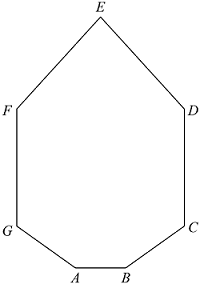# SAT PolygonsA diagonal in a polygon is defined as a segment connecting two non-adjacent vertices. How many diagonals does the figure above have?

(A) $\ \ 14$
(B) $\ \ 16$
(C) $\ \ 17$
(D) $\ \ 18$
(E) $\ \ 28$

×